## 1. Traverse Direction Computations

After angles are balanced by whatever method selected, the direction of each traverse line must be determined.

The direction of one line must be known or assumed and by using horizontal angles other successive line directions are computed. The computational process was discussed in Directions Chapter B.

## 2. Bearings From Interior Angles

Given the following traverse and horizontal angles: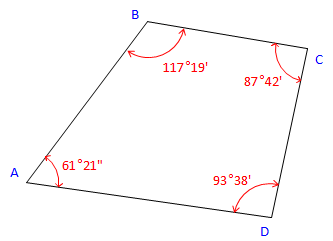Figure C-23 Bearing Traverse

Using a bearing of N 36°55' E for line AB, determine the bearings of the remaining lines clockwise around the traverse.

Many survey texts use a tabular approach to compute traverse line directions from angles. For the beginner this can be confusing and lead to erroneous directions. It helps to instead draw sketches in order to visualize the line relationships.

 At point B:Figure C-24 Label the bearing angle, 36°55', from B to A. Subtract it from 117°19'. β = 117°19' - 36°55' = 80°24'Brg BC = S 80°24' E At point C: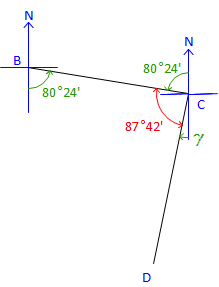Figure C-25 Label the bearing angle, 80°24', from C to B. Subtract it along with 87°42' from 180°00' to get bearing angle CD. γ = 180°00' - (80°24' + 87°42') = 11°54' Brg CD = S 11°54' W At point D: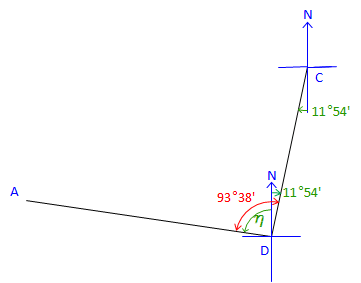Figure C-26 Label the bearing angle, 11°54', from D to C. Subtract it from 93°38' to obtain next bearing angle. &eta= 93°38' - 11°54' = 81°44'Brg DA = N 81°44' W The directions for all four traverse lines have been computed. Angles at B, C, and D have been used, but that at A has not. For a math check, use the Bearing of DA and the angle at A to compute the bearing we started with.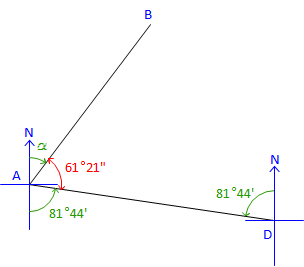Figure C-27 Label the bearing angle, 81°44', from A to D. Subtract it along with 61°21' from 180°00' to get next bearing angle. α = 180°00' - (61°21' + 81°44') = 36°55 Brg AB = N 36°55' E  check

If our computed and initial bearings for AB don’t match it means one of two things:

• there is a math error in our computations, or,
• the interior angles weren’t balanced.

For this traverse the angles sum to 360°00' so there is no angular misclosure. If our math check had failed it would have been due to a math error in our computations.

Had the angles not been balanced and if there were no math errors, the math check would be off by the angular misclosure.

Summary:

 Line Bearing AB N 36°55' E BC S 80°24' E CD S 11°54' W DA N 81°44' W

## 3. Azimuth from Interior Angles

Given the following traverse and horizontal angles:Figure C-28 Azimuth Traverse

Using a azimuth of 68°00' for line OP, determine the azimuths of the remaining lines counter-clockwise around the traverse.

 At point P:Figure C-29 Line PQ is 92°48' right of Azimuth PO Az PO is the back azimuth of Az OP Az PQ = (Az OP + 180°00') + Angle PAz PQ = (68°00' + 180°00') + 92°48' = 340°48' At point Q:Figure C-30 Line QR is 112°26' to the right from Az QP Az QP is the back azimuth of Az PQ Az QR = (Az PQ + 180°00') + Angle QAz QR = (340°48'+180°00') + 112°26' = 633°14' Normalize: Az QR = 633°14' - 360°00' = 273°14' At point R:Figure C-31 Line RO is 67°14' right from Az RQ Az RQ is the back azimuth of Az QR Az RO = (Az QR + 180°00') + Angle RAz RO  = (273°14' + 180°00') + 67°14' = 520°28' Normalize: Az RO = 520°28' - 360°00' = 160°28' The directions for all four traverse lines have been computed. Angles at P, R, and R have been used, but not the angle at O. For a math check, use Azimuth RO and the angle at O to compute the original Az OP. At point O: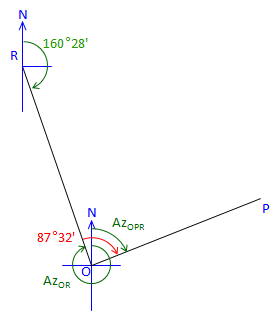Figure C-32 Line OP is 87°32' right of Az OR Az OR is the back azimuth of Az RO Az OP = (Az RO + 180°00') + Angle O Az OP = (160°28' + 180°00') + 87°32' = 428°00' Normalize: Az OP = 428°00' - 360°00' = 68°00' check!

Summary:

 Line Bearing OP 68°00' PQ 340°48' QR 273°14' RO 160°28'

There's a distinct pattern computing these azimuths:

New Az = (Previous Az + 180°00') + Angle.

This is true for a loop traverse meeting these conditions:

• Directions are counter-clockwise around the traverse, and,
• Angles are interior to the right.

What if the directions are clockwise around the traverse and interior angles counter-clockwise?

New Az = (Previous Az - 180°00') - Angle

Other patterns exist for clockwise travel with clockwise interior angles, clockwise exterior angles, counterclockwise exterior angles, etc. Rather than memorize the possible patterns, draw a sketch, and begin computing; the pattern will present itself after a few lines.

## 4. Azimuths from Deflection Angles

Deflection angles are measured traveling clockwise around a loop traverse. Starting with an azimuth of 328°58'15" for line AB, determine azimuths of the remaining lines.##### (a) Check angular misclosure.

Since this is a non-crossing loop traverse, the deflection angle sum should be ±360°00'00". Right deflection angles are positive, left are negative.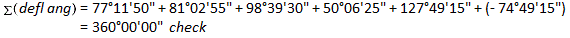There is no misclosure to distribute.

##### (b) Compute azimuths

Starting with the azimuth of line ABLine AB's extension through B has the same azimuth as line AB.

Because the deflection angle to line BC is measured from the extension of line AB, the azimuth of line BC is the azimuth of line AB plus the deflection angle:Since the azimuth exceeded 360°, it is normalized by subtracting 360° from it.

Continuing to line CD, we use the same logic: New azimuth = previous azimuth + deflection angleAnd so on...

At point DAt point EAt point FFinally, at point A compute back into the first azimuth to make sure there are no math errors:## 5. Bearings from Deflection Angles

Deflection angles are measured traveling counter-clockwise around a loop traverse. Starting with an bearing of S 18°45'30" W for line JK, determine bearings of the remaining lines.##### (a) Check angular misclosure.

Since this is a non-crossing loop traverse, the deflection angle sum should be ±360°00'00". Right deflection angles are positive, left are negative.##### (b) Compute bearings

Depending on bearing quadrants and deflection angle direction, the deflection angle might be added to or subtracted from the previous bearing angle or vice versa to compute the next bearing. While it's possible to set up some rules, it's simpler to draw a sketch to visualize the relationships and computation.

At point KLine JK's extension through K has the same bearing as line JK.

In this case, the bearing angle is computed by subtracting line JK's bearing from the deflection angle:Which puts line KL in the SE quadrant:At point LFrom the sketch, it can be seen that the sum of the previous bearing and deflection angles exceed 180° putting line LM in the NW quadrant:At point MIn this case, the previous bearing angle is subtracted from the deflection angle:At point NThis one is a little more convoluted because of the lines and angles geometry.

From the sketch:At point J

Finally, always compute back into the beginning direction as a math check.## 6. Deflection Angles from Azimuths

##### (a) Process

Because a deflection angle is the angle from the projection of the previous line to the next line, it is the difference between the azimuths of the two lines. For example, in the diagram below the deflection angle Y is the difference between the outgoing azimuth (AzYZ) and the incoming azimuth (AzXY).defl angY = AzYZ-AzXY

By subtracting the incoming azimuth from the outgoing azimuth, the correct mathematical sign is returned for the deflection angle. In the previous diagram, the deflection angle would be to the left. In the following diagram:the deflection angle is to the right.

Whether you remember to subtract the incoming from the outgoing azimuth or not, the important thing is that the deflection angle is the difference between the azimuths. As in may survey computations, a properly drawn sketch is extremely beneficial.

##### (b) Example Azimuths to Deflection AnglesAt point LAt point MAt point NAt point OLooking at the diagram, the deflection angle should be to the right so the difference should be positive.
Because the difference falls outside of the ±180°00'00" deflection angle range, add 360°00'00" to it:The is the same result if you added 360°00'00" to the outgoing azimuth before subtracting the incoming azimuth.

BTW, a deflection of 84°31'35"R is the same as a 275°28'25"L deflection.

At point PMath check

For a non-crossing loop traverse, the deflection angle sum should be ±360°## 7. Angles from Bearings

Given bearings going counter-clockwise around a loop traverse, compute the interior angles at each point.Unlike computing directions from angles, it doesn't matter where to start or in what order or direction to compute the interior angles.
Drawing sketches helps visualize how to combine the bearings at a point to compute the angle.

At point RAt point SAt point TAt point UAt point VAt point WMath check## 8. Summary

Converting between angles and directions isn't difficult, but there are a few things someone new to the computations needs to watch out for.

This usually happens when the sketch isn't correctly drawn. In the diagram below, the surveyor assumed line JK was in the NE quadrant.The bearing angle is the difference between the back bearing and the angle J. Based on his sketch, the surveyor recorded the bearing JK as S 8°19'15" E while it really is N 08°19'15" W. While a sketch helps, an incorrectly drawn one can lead to wrong answers.

#### (2) Bearing angle exceeds 90°

In this example, the surveyor computed the bearing angle as the sum of the back bearing angle and angle M, recording the bearing of line MN as N 91°05'45" EWhen a bearing angle exceeds 90°,  the line crosses into the adjacent quadrant. Line MN's correctly written bearing  is S 88°54'15" E

#### (3) Confusing Directions and Back Directions

A number of Surveying textbooks use a tabular method to compute directions from angles. These are based on a systematic computation process like the Azimuth from Deflection Angles example in this section. While efficient, it may require flipping back and forth between forward and back directions depending on the direction type, which can lead to errors (like being exactly 180° off or flipped bearing quadrant). It requires the surveyor understand the systematic process that will be applied. Consider the Azimuth from Deflection Angles example in this section: it was for traveling counter-clockwise around the traverse. What changes in the computation process if travel ran clockwise?

The biggest drawback to a tabular method is there is no visualization. A sketch helps organize directions and angles allowing the surveyor to see the relationships. It's easier to spot a back bearing error on a sketch than in a table. Plus, as shown in the Azimuth from Deflection Angles example, using sketches for the first few computations helps identify the systematic process which can simplify subsequent calculations.

#### (4) Using the East-West line

Directions are always referenced to a meridian, a North-South line. When performing calculations, angles should never be computed from the East-West line as it may lead to confusion. Students working with directions early on tend to break up angles into smaller parts, often computing parts from the East-West line. For example, in the following sketch, an intermediate calculation may be:Bearing line RS would is N 70°19'30" W from:However, some will report it as N 19°40'30" W by mistake or even W 19°40'30" N.
Using the East-West line adds another layer of calculations and potential error.

#### (5) Math mistakes

Most errors are mistakes made when adding or subtracting angles. This is generally because angles are a mixed unit system, deg-min-sec. Calculators which have built-in degree conversion or direct deg-min-sec manipulation capabilities help reduce the problem (providing the user knows how to use the calculator). In manual calculations, subtraction errors are generally more prevalent than addition errors, eg, 180°00'000" - 50°25'30"= 130°35'30".

For each example problem presented here, we identified and applied a math check. If a math check fails, it won't tell us where exactly the error(s) occurred,  but it will let us know there is an error.

Always, always, apply an applicable math check.

## 9. Self-Study Problems

### Problem (1)

Using the balanced angles shown below, determine the direction of each line traveling counter-clockwise around the traverse.
Perform appropriate math checks(s).Point Angle (right) A 89°40'00" B 114°50'05" C 115°39'55" D 80°06'10" E 139°43'50" Brng AB = S 54°24'20"W

### Problem (2)

Using the balanced angles shown below, determine the direction of each line traveling clockwise around the traverse.
Perform appropriate math checks(s).Point Angle (left) H 128°31'38" J 67°51'02" K 127°24'40" L 49°14'35" M 282°41'22" N 64°16'43" Azimuth HJ = 279°16'45"

### Problem (3)

Using the interior angles shown, compute line bearings going counter-clockwise around the traverse. Identify and perform a math check.Bearing of line TU is  S 79°46'35" E.

### Problem (4)

Given the information below, compute line bearings going clockwise around the traverse.### Set b. Angles from Directions

#### Problem (1)

Compute deflection angles at points B through F for the link traverse below. Identify and perform a math check.